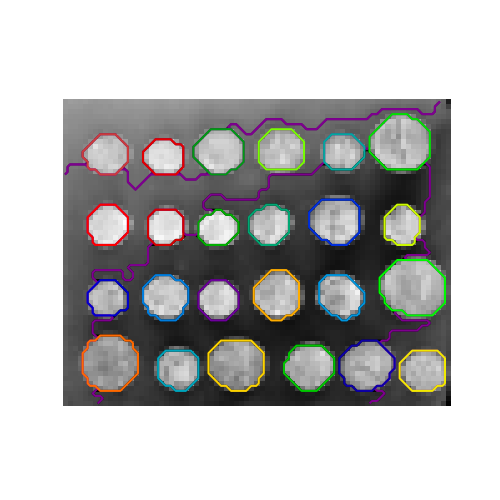# A demo of structured Ward hierarchical clustering on an image of coins¶

Compute the segmentation of a 2D image with Ward hierarchical clustering. The clustering is spatially constrained in order for each segmented region to be in one piece.

# Author : Vincent Michel, 2010
#          Alexandre Gramfort, 2011


## Generate data¶

from skimage.data import coins

orig_coins = coins()


Resize it to 20% of the original size to speed up the processing Applying a Gaussian filter for smoothing prior to down-scaling reduces aliasing artifacts.

import numpy as np
from scipy.ndimage import gaussian_filter
from skimage.transform import rescale

smoothened_coins = gaussian_filter(orig_coins, sigma=2)
rescaled_coins = rescale(
smoothened_coins,
0.2,
mode="reflect",
anti_aliasing=False,
)

X = np.reshape(rescaled_coins, (-1, 1))


## Define structure of the data¶

Pixels are connected to their neighbors.

from sklearn.feature_extraction.image import grid_to_graph

connectivity = grid_to_graph(*rescaled_coins.shape)


## Compute clustering¶

import time as time

from sklearn.cluster import AgglomerativeClustering

print("Compute structured hierarchical clustering...")
st = time.time()
n_clusters = 27  # number of regions
ward = AgglomerativeClustering(
)
ward.fit(X)
label = np.reshape(ward.labels_, rescaled_coins.shape)
print(f"Elapsed time: {time.time() - st:.3f}s")
print(f"Number of pixels: {label.size}")
print(f"Number of clusters: {np.unique(label).size}")

Compute structured hierarchical clustering...
Elapsed time: 0.138s
Number of pixels: 4697
Number of clusters: 27


## Plot the results on an image¶

Agglomerative clustering is able to segment each coin however, we have had to use a n_cluster larger than the number of coins because the segmentation is finding a large in the background.

import matplotlib.pyplot as plt

plt.figure(figsize=(5, 5))
plt.imshow(rescaled_coins, cmap=plt.cm.gray)
for l in range(n_clusters):
plt.contour(
label == l,
colors=[
plt.cm.nipy_spectral(l / float(n_clusters)),
],
)
plt.axis("off")
plt.show()Total running time of the script: ( 0 minutes 0.517 seconds)

Gallery generated by Sphinx-Gallery